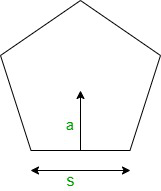Open in App
Not now

# How to find the Area of Pentagon?

• Last Updated : 01 Feb, 2022

Geometry is a branch of mathematics that is all about the study of shapes. In general, we study two types of shapes in geometry one is the flat shape and the other solid shapes. Flat shapes are plotted on flat surfaces which are two-dimensional and solid shapes are three-dimensional. Geometry also deals with the parameters of these shapes. It gives standard formulas for determining their parameters like area, perimeter, volume, etc.

The given article deals with one of the flat shapes pentagons. It gives a brief description of the pentagon and its properties. The article explains the area formula of a pentagon along with some sample problems for better understanding.

### What is a Pentagon?

The word pentagon denotes ‘five angles’ as “Penta” means five and “gon” means angles. It is a geometrical shape having five sides and five angles. It is a five-sided self-intersecting polygon.  And, the sum of all the interior angles of a polygon measures to be 540 degrees.

Summing up, a pentagon is a five-sided, two-dimensional geometrical shape whose interior angles sum up to be 540 degrees.

Properties of a pentagon

• A pentagon has five sides.
• It has 5 diagonals meeting at the same point.
• The interior angles of a pentagon measure to be 72 degrees.
• the exterior angles of a pentagon measure to be 108 degrees.

### How To find the Area of Pentagon?

Using Apothem Length

The area of a pentagon is determined by its side and apothem length. The formula of the area of a pentagon is derived by multiplying any side and apothem length by 5/2.

Mathematically the formula is given by

Area of pentagon(A)=(5/2) s × a

where,

s is the side

and a is apothem lengthPentagon

For example:

If a side of a pentagon is 12cm and its apothem length is 6cm, the area of the pentagon can be determined by

Area of pentagon= (5/2) × side × apothem length

= (5/2) × 12 × 6

= 180cm2

Using only Side Length

The area of the pentagon can also be calculated only by using the length. Here, to calculate the area of the given pentagon by using only the side length following formula is usedwhere,

s is the side length

For example:

If a pentagon is given having side length of 5cm, the area of the pentagon can be determined by

Area of pentagon === 43.01cm2

### Sample Problems

Problem 1. Find the area of a pentagon with a side 5cm and apothem length 4cm.

Solution:

Given

Side of pentagon = 5cm

apothem length = 4cm

We have,

Area = (5/2) × s × a

=>A = (5/2) × 5 × 4

=>A = 50cm2

Problem 2. Find the area of a pentagon with a side 12cm and apothem length of 6cm.

Solution:

Given

Side of pentagon = 12cm

apothem length = 6cm

We have,

Area = (5/2) × s × a

=>A = (5/2) × 12 × 6

=>A = 180cm2

Problem 3. Find the area of a pentagon with a side 12cm and apothem length of 4cm.

Solution:

Given

Side of pentagon = 12cm

apothem length = 4cm

We have,

Area = (5/2) × s × a

=>A = (5/2) × 12 × 4

=>A = 120cm2

Problem 4. Find the area of a pentagon with a side 10cm and apothem length of 5cm.

Solution:

Given

Side of pentagon = 10cm

apothem length = 5cm

We have,

Area = (5/2) × s × a

=>A = (5/2) × 10 × 5

=>A = 125cm2

Problem 5. Find the area of a pentagon with a side 8cm and apothem length of 5cm.

Solution:

Given

Side of pentagon = 8cm

apothem length = 5cm

We have,

Area = (5/2) × s × a

=>A = (5/2) × 8 × 5

=>A = 100cm2

Problem 6. Find the area of a pentagon with a side length of 4cm.

Solution:

Given

Side length of pentagon is 4cm

We have,

Area of pentagon ==>=>27.52cm2

Problem 7. Find the area of a pentagon with a side length of 6cm.

Solution:

Given,

Side length of pentagon is 6cm.

We have,

Area of pentagon ==>=>61.93cm2

My Personal Notes arrow_drop_up
Related Articles